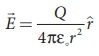Home | | Physics 12th Std | Electrostatics: Summary and Formulas

# Electrostatics: Summary and Formulas

Like charges repel and unlike charges attract

SUMMARY

·        Like charges repel and unlike charges attract

·        The total charge in the universe is conserved

·        Charge is quantized. Total charge in an object q = ne where n = 0,1,2,3… and e is electron charge.

·        Coulomb’s law in vector form:(r ^ is unit vector along joining q1, q2)

·        For continuous charge distributions, integration methods can be used.

·        Electrostatic force obeys the superposition principle.

·        Electric field at a distance r from a point charge:·        Electric field lines starts at apositive charge and end at a negative charge or at infinity

·        Electric field due to electric dipole at points on the axial line :·        Electric field due to electric dipole at points on the equatorial line:·        Torque experienced by a dipole in a uniform electric field:·        Electrostatic potential at a distance r from the point charge:·        Electrostatic potential due to an electric dipole:·        The electrostatic potential is the same at all points on an equipotential surface.

·        The relation between electric field and electrostatic potential:·        Electrostatic potential energy for system of charges is equal to the work done to arrange the charges in the given configuration.

·        Electrostatic potential energy stored in a dipole system in a uniform electric field:·        The total electric flux through a closed surface : ΦE = Q/ε0  where Q is the net charge enclosed by the surface

·        Electric field due to a charged infinite wire·        Electric field due to a charged infinite plane :·        Electric field inside a charged spherical shell is zero. For points outside:·        Electric field inside a conductor is zero. The electric field at the surface of the conductor is normal to the surface and has magnitude E = σ/ε0 .

·        The surface of the conductor has the same potential, at all points on the surface.

·        Conductor can be charged using the process of induction.

·        A dielectric or insulator has no free electrons. When an electric field is applied, the dielectric is polarised.

·        Capacitance is given by C = Q/V .

·        Capacitance of a parallel plate capacitor: C = εA / d

·        Electrostatic energy stored in a capacitor: U = 1/2 CV2

·        The equivalent capacitance for parallel combination is equal to the sum of individual capacitance of capacitors.

·        For a series combination: The inverse of equivalent capacitance is equal to sum of inverse of individual capacitances of capacitors.

·        The distribution of charges in the conductors depends on the shape of conductor. For sharper edge, the surface charge density is greater. This principle is used in the lightning arrestor

·        To create a large potential difference, a Van de Graaff generator is usedTags : Physics , 12th Physics : Electrostatics
Study Material, Lecturing Notes, Assignment, Reference, Wiki description explanation, brief detail
12th Physics : Electrostatics : Electrostatics: Summary and Formulas | Physics